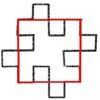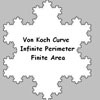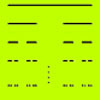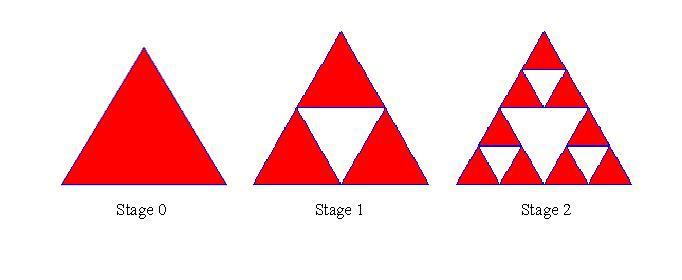#### You may also like### Squareflake

A finite area inside and infinite skin! You can paint the interior of this fractal with a small tin of paint but you could never get enough paint to paint the edge.### Von Koch Curve

Make a poster using equilateral triangles with sides 27, 9, 3 and 1 units assembled as stage 3 of the Von Koch fractal. Investigate areas & lengths when you repeat a process infinitely often.### How Many Elements Are There in the Cantor Set?

This article gives a proof of the uncountability of the Cantor set.

# Sierpinski Triangle

##### Age 16 to 18Challenge Level

The diagram shows the first three shapes in a sequence that goes on for ever and, in the limit, gives the Sierpinski triangle.

How many red triangles are there at Stage $n$? If the area of the red triangle at Stage $0$ is $1$, what is the total area of the red triangles at Stage $n$? How many white triangles are there at Stage $n$, that is how many triangles altogether have been removed? What is the total area of the triangles removed? What happens to these areas as $n \to \infty$? What is the dimension of this fractal?The sequence starts with a red triangle. Each triangle in the sequence is formed from the previous one by removing, from the centres of all the red triangles, the equilateral triangles formed by joining the midpoints of the edges of the red triangles. If this process is continued indefinitely it produces a fractal called the Sierpinski triangle.

Now you are going to work out the dimension of this fractal. If you imagine moving about within this fractal then you have more choice of direction in which to go than if you were on a line and less choice of direction than in a square so you would expect the dimension of the fractal to be between $1$ and $2$.

We can break up the Sierpinski triangle into 3 self similar pieces $(n=3)$ then each can be magnified by a factor $m=2$ to give the entire triangle.

The formula for dimension $d$ is $n = m^d$ where $n$ is the number of self similar pieces and $m$ is the magnification factor.

#### NOTES AND BACKGROUND

If you break a line of length $1$ into self similar bits of length ${1\over m}$ there are $m^1$ bits and we say the dimension of the line is $1$.

If you break up a square of side $1$ into self similar squares with edge ${1\over m}$ then there are $m^2$ smaller squares and we say the dimension is $2$.

If you break up a cube of side $1$ into self similar cubes with edge ${1\over m}$ then there are $m^3$ smaller cubes and we say the dimension is 3.

In each case we say the magnification factor is $m$ meaning that we have to scale the lengths by a factor of $m$ to produce the original shape. So the formula is: $$\text{number of bits} = \text{(magnification factor})^d$$ where d is the dimension, i.e. $n = m^d$.